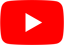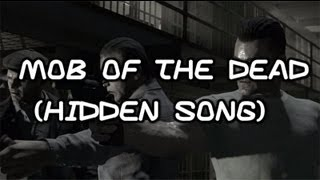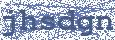Where Are We Going
Elena Siegman

## ELENA SIEGMAN

Where Are We Going Lyrics

Where are we going from here
Where do we go
Are we all blinded by fear

How do we know, How do we know
Where do we go. Where do we go
How do we know, where do we go
Where are we going from here
How do we know, where do we go

Where are we going from here
Do we let go of all we know?
Are we all blinded from fear
Where do we go when we let go

I feel I'm falling from here
Dont let me go
Is it the calling?
we hear, we hear
how do, how do
we know...Hottest Lyrics with Videos